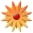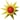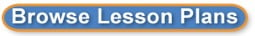# Introducing Multiplication

Page Views: 40952

Email This Lesson Plan to Me
Overall Rating:(5.0 stars, 1 ratings)

 Keywords: Multiplication, Repeated Addition, Manipulatives Subject(s): Math Grades 2 through 4 School: Chimneyrock Elementary School, Cordova, TN Planned By: Amanda Loyacano Original Author: Amanda Loyacano, Cordova
Lesson Sequence  Introductory (This will be the students first experience with multiplication this school year.)

Assessment
-Pre: At the beginning of the year, students were given a second grade skills test as well as the ThinkLink test of second grade skills. I have used both of these test data to obtain information about the level of student understanding of multiplication.
-Post: Students will be assessed informally during the lesson. Later in the week they will receive a formal assessment to determine their level of skill in multiplication concepts.

Instructional Objectives
TLW understand the concepts that multiplication is repeated addition.
TLW use concrete patterns to predict a product.
SPI: 3.1.spi.16 Use the multiplication facts 0, 1, 2, 5, and 10 efficiently & accurately.

Procedure - Anticipatory Set
Two volunteers will be asked to come to the front and hold out their hands, palms up. Next, I will count out 2 counters into each students hand. Then, I will ask the students how they can find the total number of counters in the four hands. This discussion will lead into the actual lesson.

Procedure  Multiplication Introduction
For the discussion, some ideas they may come up with are: counting the counters by ones (1+1+1+1+1+1+1+1=8), counting the counters by twos (2 + 2 + 2 + 2 = 8), etc. Counting by 2s is the goal for them to recognize.
Next, I will show students how we can write the repeated addition sentence as a multiplication sentence, 2 + 2 + 2 + 2 = 8 is the same as 4 x 2 = 8 (read as 4 times 2 equals 8 & 4 groups off 2 equals 8 counters total). We will then discuss which way is easier to write, repeated addition or multiplication so that students can better understand why we use multiplication.
Finally, I will ask students how they could record this example on graph paper. I will use a transparency of graph paper to test out their ideas.

Procedure  Supervised Practice
First, I will pass out packs of 1-8 index cards, counters, and graph paper to each group of students. Next, we will do an example together. A student will pick a number between 1 and 10. For example, if the number is 3 then each group will place 3 counters on each of their cards, write a repeated addition problem, a multiplication problem, and show it on the graph paper. So, depending on how many index cards they have, each group will arrive at a different multiplication problem. I will write their multiplication sentences on the board so that we can keep a chart of what we have found.
We will share after each example and observe what patterns we notice. We will do this example several times using different numbers with students gaining independence as we go along. If there is time, groups may swap the number of index cards they have to try out another group of numbers.

Closure
To wrap up the lesson we will discuss the patterns that students found. Also, I will pose the question of what would the product be if we had zero index cards because 0s will be the first group of multiplication facts that we will learn.

Meeting Individual Students Needs
* I will observe students level of understanding through questioning & monitoring of progress during the activity:
-Reteach/Remediation: I will use guided questions to help students discover how repeated addition is the same as multiplication. How many groups of you have? How many are in each group? What is the total? What addition and multiplication sentences could we use to show this? Also, I will use consistent language: 3 groups of 2 added together equals 6, just as 3groups times 2 groups equals 6.
-Enrichment: I will give higher-level groups of students a larger number of index cards or an amount that they cannot easily count by (4 & 6) compared to groups that may have more difficulty who will receive the number of cards that are easier to count by (1, 2, 3, & 5).
-Independent Practice: Students will independently complete examples on their own using the index cards, counters, and graph paper. Sheets will be collected at the end of the lesson to monitor their level of understanding.

Materials Use
Overhead & Graph Paper Transparency To show students how to draw examples
Index cards To show groups
Counters To show individual parts of the group
Graph Paper To show pictures & number sentences

 Comments This is a great way for kinesthetic & visual learners to understand basic multiplication concepts. Follow-Up Have students create their own multiplication sentences and picture arrays. Materials: Worksheets Other Items: 1 Bag of Math Counters, \$10.00 each, total of \$10.001 Pack of Large Index Cards, \$3.00 each, total of \$3.001 Pack of Graph Paper, \$2.00 each, total of \$2.00